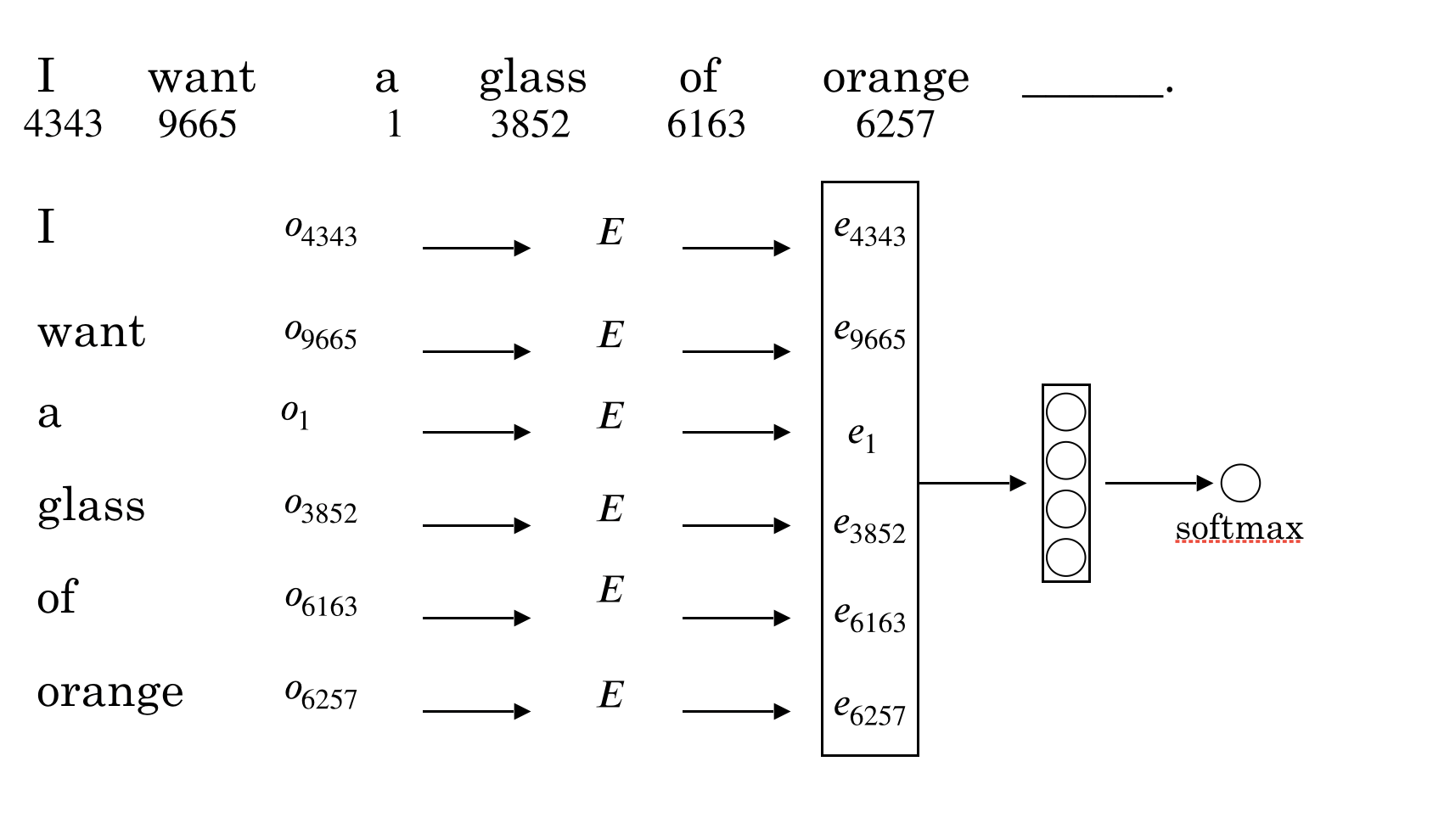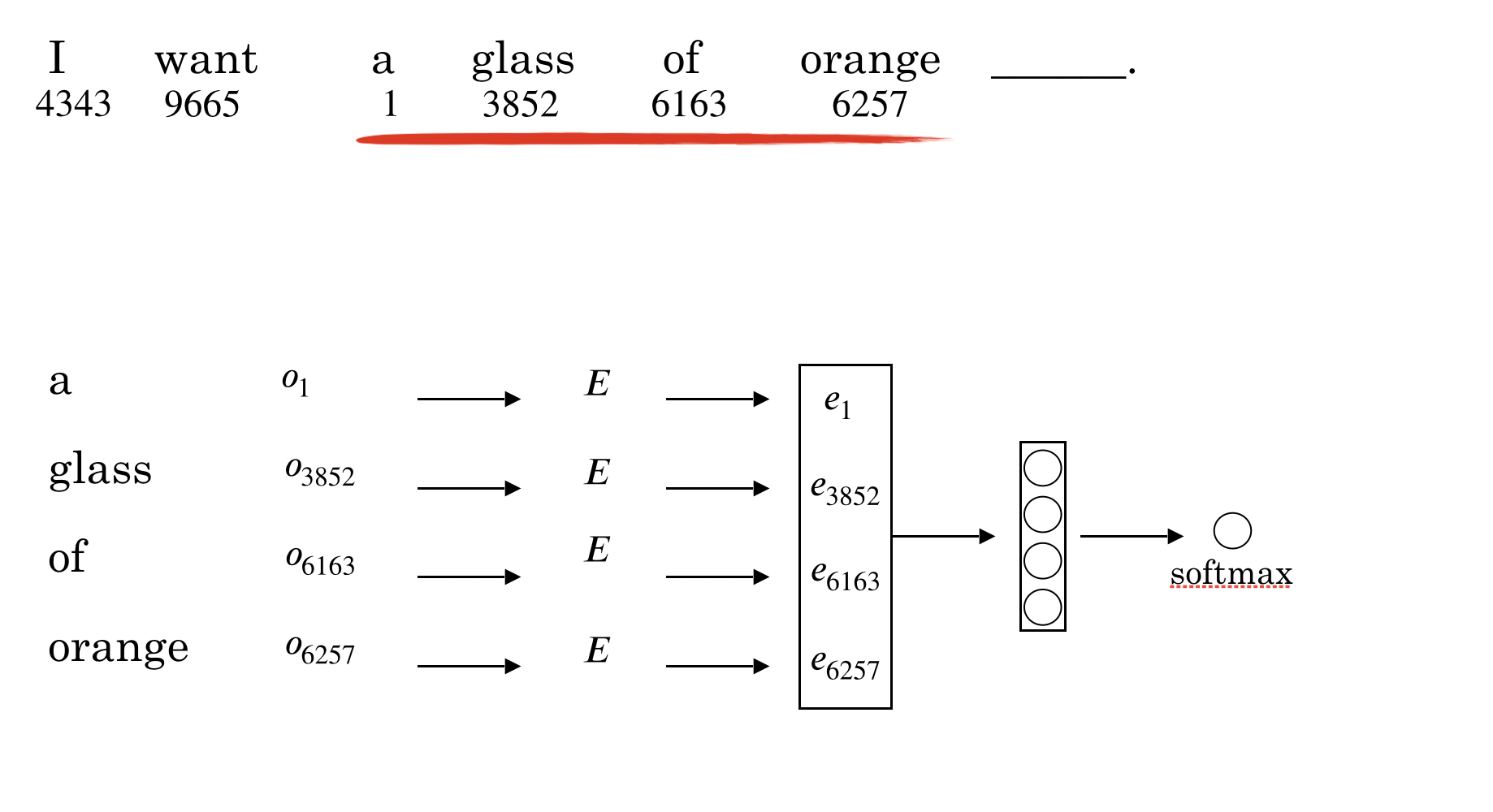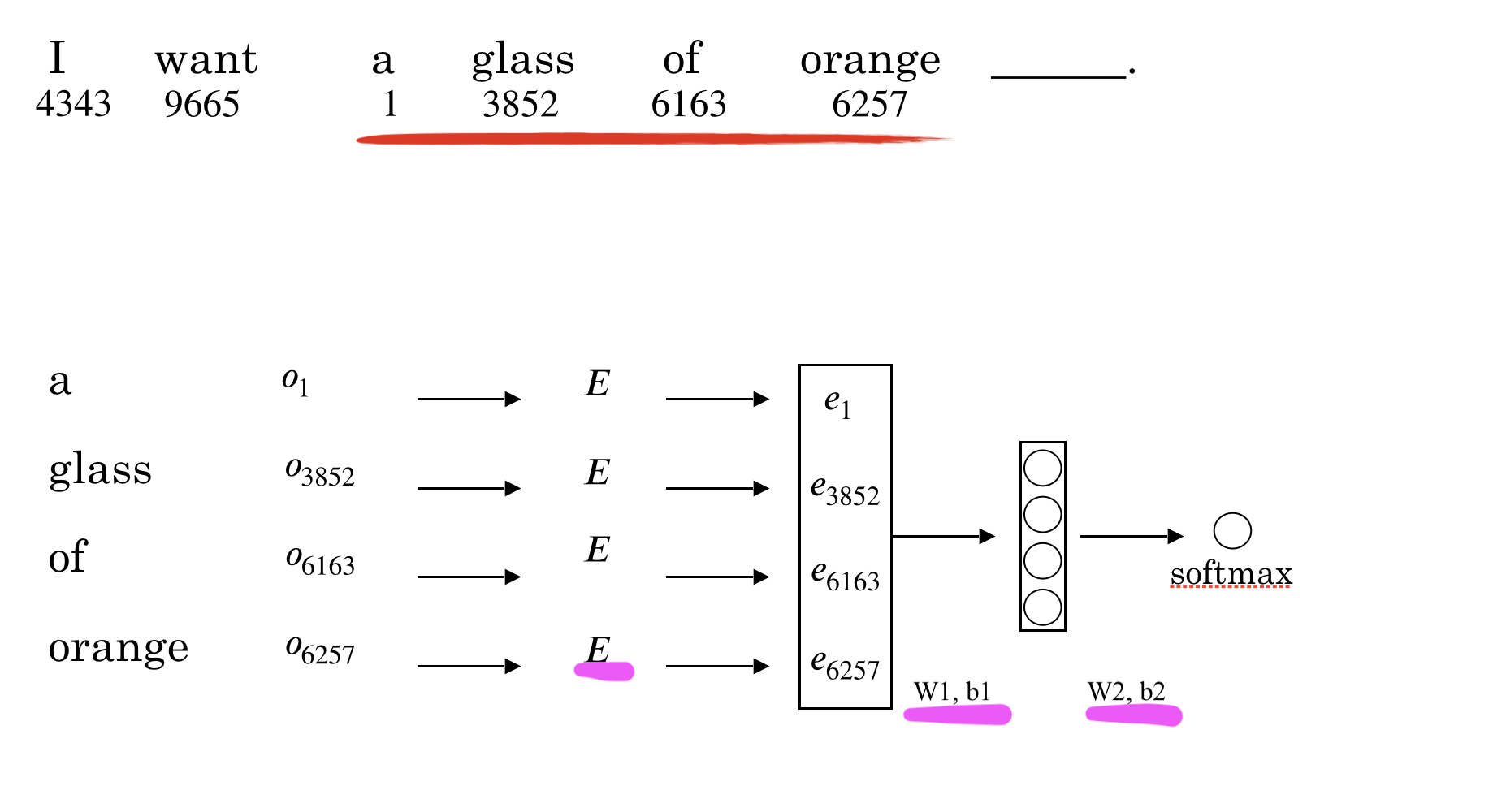2020-06-22

# Learn word embedding1# Learn word embedding# Learn word embedding# Other context/target pairs

I want a glass of orange juice to go alone with my cereal.

Context:

• Last 4 words: a glass of orange $$\longrightarrow$$ ?
• 4 words on the left & right: a glass of orange ? to go alone with
• Last 1 word: orange $$\longrightarrow$$ ?
• Nearby 1 word (skip gram model): glass $$\longrightarrow$$ ?

# Word2Vec2: Skip-grams

Come up with a few context to target pairs to create our supervised learning problem

Rule: randomly pick a word as context word; randomly pick another word within some window ($$\pm 3$$) as target word

I want a glass of orange juice to go along with my cereal.

Context Target
orange juice
orange glass
orange go

# Word2Vec: Model

Context $$\longrightarrow$$ Target
c (“orange”) $$\longrightarrow$$ t (“juice”)

$O_c \rightarrow E \rightarrow e_c \rightarrow softmax \rightarrow \hat{y}$

$Softmax: p(t|c)= \frac{e^{\theta_t^Te_c}}{\Sigma_{j=1}^{10,000}e^{\theta_j^Te_c}}$

$L(\hat{y}, y)=-\Sigma_{i=1}^{10,000} y_i log\hat{y}_i$

• Parameters:
• $$\theta_t$$:parameter associated with output t
• E: embedding matrix

# Problems with softmax classification

1. Bengio et. al., 2003, A neural probabilistic language model

2. Mikolov et. al., 2013. Efficient estimation of word representations in vector space